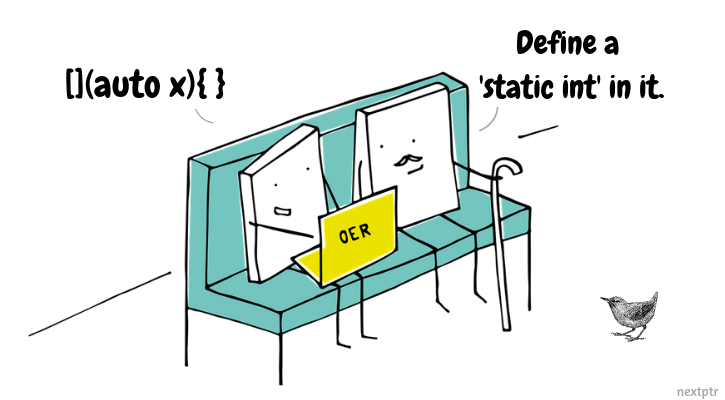# Generic lambda with static local variableA lambda with `auto` parameter(s) is called generic lambda. Consider a simple generic lambda with a static local variable defined as:

``````auto lambda = []( auto x ) {
static int y = 0;   // static local
return ++y + x;
};
``````

What would be the result of calling above lambda three times as:

``````std::cout << lambda(1) << " "
<< lambda(1.0) << " "
<< lambda(1) << std::endl;
``````

Choose the correct answer below (check Explanations for explanation of answer):